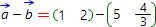# Vectors difference onlineVector difference is the vector which coordinates can be calculated by subtraction of corresponding coordinates of the vectors to subtract. If vectors are represented in geometrical form, their difference can be found using following picture:Therefore, to find vector difference represented in geometrical form, one should match their initial point. Then their difference is the vector whose initial point matches the final point of the vector to subtract ( b ), and the final point - matches with final point of the vector to subtract from ( a ).

This online calculator can be used to find subtraction of the vectors represented in coordinate form or with coordinates of their initial and final points. Step by step solution is also available for free.

Vectors subtraction calculatorby:by:= { }= { }